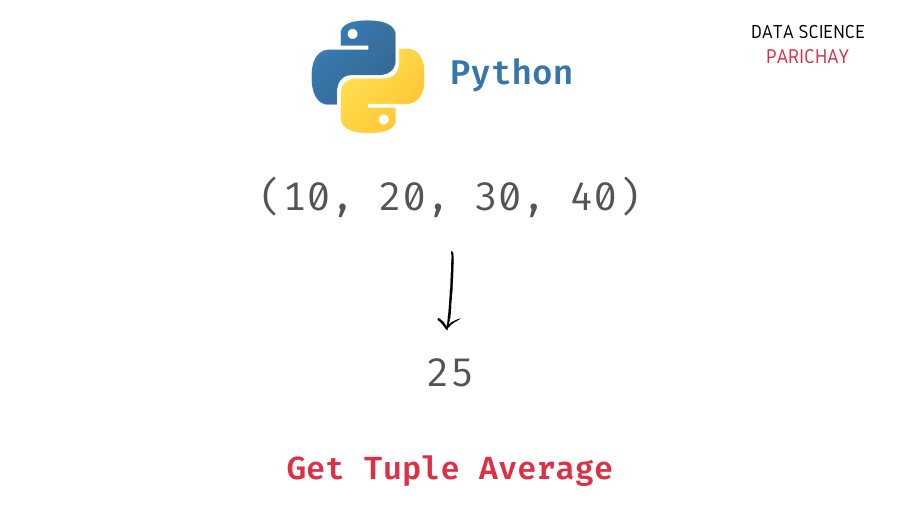# Get Average of Elements in a Python Tuple

Tuples are a data structure used in Python to store an ordered collection of objects. When working with tuples, it might be handy to know how to quickly compute the mean. In this tutorial, we will look at how to get the average of a tuple with numeric values in Python.

You can use a combination of the Python built-in `sum()` and `len()` functions to get the average of a tuple. Alternatively, you can also use methods from libraries like `statistics`, `numpy`, etc.

Let’s look at these methods with the help of some examples.

To get the tuple mean, compute the tuple sum using the `sum()` function and divide it by the length of the tuple (which you can get using the `len()` function).

Here’s an example.

```# create a tuple
t = (10, 20, 30, 40)
# get average of tuple values
print(sum(t)/len(t))```

Output:

`25.0`

We get the average of the tuple containing the values 10, 20, 30, and 40 as 25.

You can also compute the mean of a tuple directly using off-the-shelf methods from some libraries like `statistics` and `numpy`.

📚 Data Science Programs By Skill Level

Introductory

Intermediate ⭐⭐⭐

🔎 Find Data Science Programs 👨‍💻 111,889 already enrolled

Disclaimer: Data Science Parichay is reader supported. When you purchase a course through a link on this site, we may earn a small commission at no additional cost to you. Earned commissions help support this website and its team of writers.

You can use the `statistics` standard library’s `mean()` function to get the mean of a tuple. Pass the tuple as an argument to the function.

Here’s an example.

```import statistics

# create a tuple
t = (10, 20, 30, 40)
# get average of tuple values
print(statistics.mean(t))```

Output:

`25`

We get the same result as above.

For more on the `statistics` library, refer to its documentation.

You can also use the `numpy` library to get the average of values in a tuple. Pass the tuple as an argument to the library’s `mean()` function.

Let’s look at an example.

```import numpy as np

# create a tuple
t = (10, 20, 30, 40)
# get average of tuple values
print(np.mean(t))```

Output:

`25.0`

We get 25 as the mean, the same result that we got with the methods above.

You might also be interested in –

•